## Introduction

Missing data can be a not so trivial problem when analysing a dataset and accounting for it is usually not so straightforward either.

If the amount of missing data is very small relatively to the size of the dataset, then leaving out the few samples with missing features may be the best strategy in order not to bias the analysis. However leaving out available datapoints deprives the data of some amount of information and depending on your situation. You may want to look for other fixes before wiping out potentially useful data points from your dataset.

While some quick fixes such as mean-substitution may be fine in some cases, these approaches usually introduce bias into the data.

The MICE package in R, helps you imputing missing values with plausible data values. These plausible values are drawn from a distribution specifically designed for each missing datapoint.

Impute missing values using a the airquality dataset. To simplify things, some data will be removed.

data <- airquality
data[4:10,3] <- rep(NA,7)
data[1:5,4] <- NA

Replacing categorical variables is usually not advisable. Some common practice include replacing missing categorical variables with the mode of the observed ones. However, it is questionable whether it is a good choice. Even though in this case no data points are missing from the categorical variables, we remove them from our dataset (we can add them back later if needed) and take a look at the data using summary().

data <- data[-c(5,6)]
summary(data)
##      Ozone           Solar.R           Wind             Temp
##  Min.   :  1.00   Min.   :  7.0   Min.   : 1.700   Min.   :57.00
##  1st Qu.: 18.00   1st Qu.:115.8   1st Qu.: 7.400   1st Qu.:73.00
##  Median : 31.50   Median :205.0   Median : 9.700   Median :79.00
##  Mean   : 42.13   Mean   :185.9   Mean   : 9.806   Mean   :78.28
##  3rd Qu.: 63.25   3rd Qu.:258.8   3rd Qu.:11.500   3rd Qu.:85.00
##  Max.   :168.00   Max.   :334.0   Max.   :20.700   Max.   :97.00
##  NA's   :37       NA's   :7       NA's   :7        NA's   :5

Apparently Ozone is the variable with the most missing datapoints. Below we are going to dig deeper into the missing data patterns.

## Quick classification of missing data

There are two types of missing data:

• MCAR: missing completely at random. This is the desirable scenario in case of missing data.
• MNAR: missing not at random. Missing not at random data is a more serious issue and in this case it might be wise to check the data gathering process further and try to understand why the information is missing. For instance, if most of the people in a survey did not answer a certain question, why did they do that? Was the question unclear?

Assuming data is MCAR, too much missing data can be a problem too. Usually a safe maximum threshold is 5% of the total for large datasets. If missing data for a certain feature or sample is more than 5% then you probably should leave that feature or sample out. We therefore check for features (columns) and samples (rows) where more than 5% of the data is missing using a simple function:

pMiss <- function(x){sum(is.na(x))/length(x)*100}
apply(data,2,pMiss)
##     Ozone   Solar.R      Wind      Temp
## 24.183007  4.575163  4.575163  3.267974
apply(data,1,pMiss)
##     25  25  25  50 100  50  25  25  25  50  25   0   0   0   0   0   0
##     0   0   0   0   0   0   0  25  25  50   0   0   0   0  25  25  25
##    25  25  25   0  25   0   0  25  25   0  25  25   0   0   0   0   0
##    25  25  25  25  25  25  25  25  25  25   0   0   0  25   0   0   0
##     0   0   0  25   0   0  25   0   0   0   0   0   0   0  25  25   0
##     0   0   0   0   0   0   0   0   0   0  25  25  25   0   0   0  25
##   25   0   0   0  25   0   0   0   0   0   0   0  25   0   0   0  25
##    0   0   0   0   0   0   0   0   0   0   0   0   0   0   0   0   0
##    0   0   0   0   0   0   0   0   0   0   0   0   0  25   0   0   0

We see that Ozone is missing almost 25% of the datapoints, therefore we might consider either dropping it from the analysis or gather more measurements. The other variables are below the 5% threshold so we can keep them. As far as the samples are concerned, missing just one feature leads to a 25% missing data per sample. Samples that are missing 2 or more features (>50%), should be dropped if possible.

## Using mice for looking at missing data pattern

The mice package provides a helpful function md.pattern() providing a better understanding of the pattern of missing data.

library(mice)
md.pattern(data)
##     Temp Solar.R Wind Ozone
## 104    1       1    1     1  0
##  34    1       1    1     0  1
##   4    1       0    1     1  1
##   3    1       1    0     1  1
##   3    0       1    1     1  1
##   1    1       0    1     0  2
##   1    1       1    0     0  2
##   1    1       0    0     1  2
##   1    0       1    0     1  2
##   1    0       0    0     0  4
##        5       7    7    37 56
• 104 samples are complete
• 34 samples miss only the Ozone measurement
• 4 samples miss only the Solar.R value

A perhaps more helpful visual representation can be obtained using the VIM package as follows:

library(VIM)
aggr_plot <- aggr(data, col=c('navyblue','red'), numbers=TRUE, sortVars=TRUE,
labels=names(data), cex.axis=.7, gap=3, ylab=c("Histogram of missing data","Pattern"))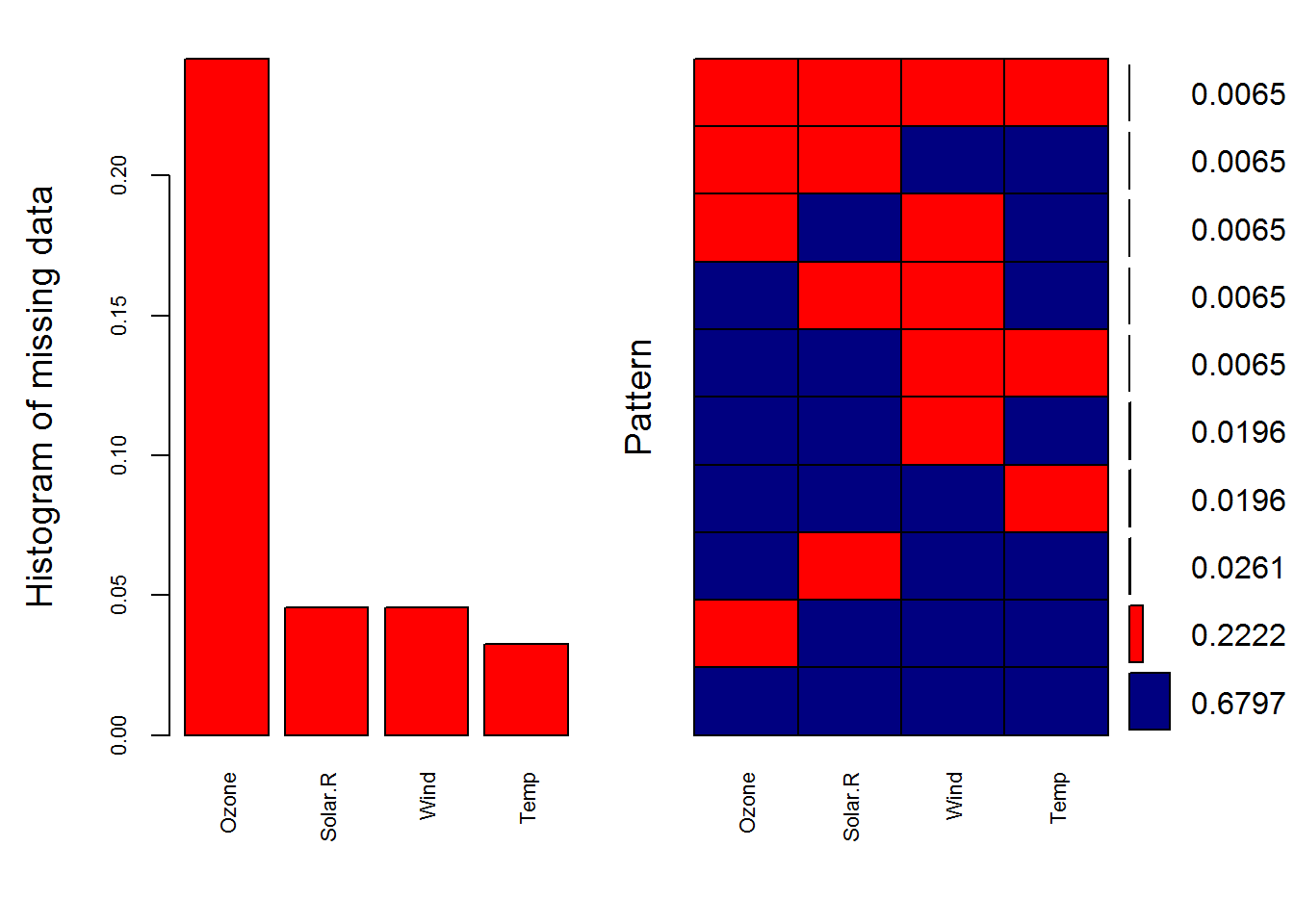##
##  Variables sorted by number of missings:
##  Variable      Count
##     Ozone 0.24183007
##   Solar.R 0.04575163
##      Wind 0.04575163
##      Temp 0.03267974

The plot helps us understand:

• almost 70% of the samples are not missing any information
• 22% are missing the Ozone value
• the remaining ones show other missing patterns.

Another visual approach is a special box plot:

marginplot(data[c(1,2)])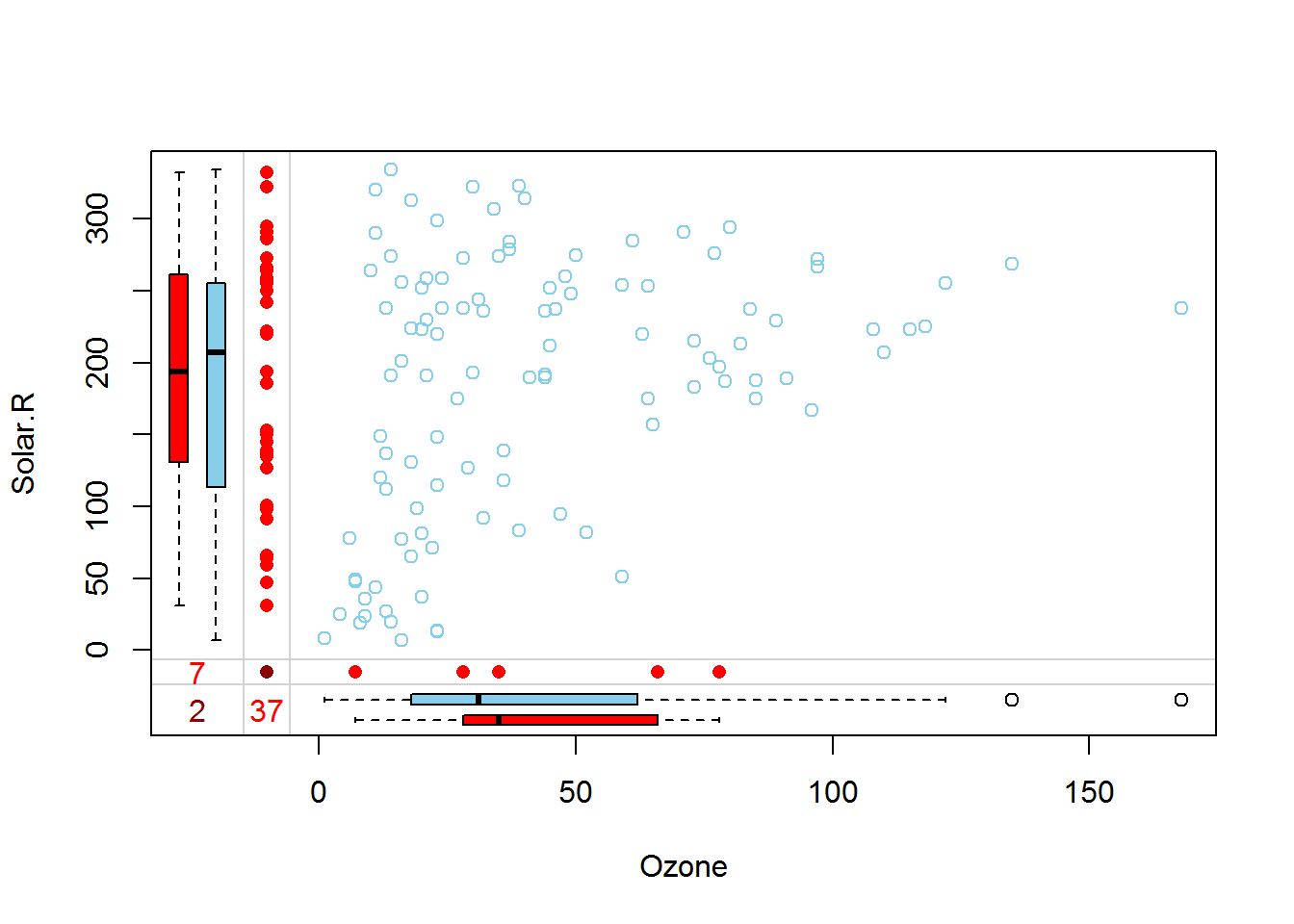We are constrained at plotting 2 variables at a time only, but we can still collect some interesting insights.

The red box plot on the left shows the distribution of Solar.R with Ozone missing while the blue box plot shows the distribution of the remaining datapoints. Likewhise for the Ozone box plots at the bottom of the graph.

If our assumption of MCAR data is correct, then we expect the red and blue box plots to be very similar.

## Imputing the missing data

The mice() function takes care of the imputing process:

library(mice)
tempData <- mice(data,m=5,maxit=50,meth='pmm',seed=500)
summary(tempData)
## Multiply imputed data set
## Call:
## mice(data = data, m = 5, method = "pmm", maxit = 50, seed = 500)
## Number of multiple imputations:  5
## Missing cells per column:
##   Ozone Solar.R    Wind    Temp
##      37       7       7       5
## Imputation methods:
##   Ozone Solar.R    Wind    Temp
##   "pmm"   "pmm"   "pmm"   "pmm"
## VisitSequence:
##   Ozone Solar.R    Wind    Temp
##       1       2       3       4
## PredictorMatrix:
##         Ozone Solar.R Wind Temp
## Ozone       0       1    1    1
## Solar.R     1       0    1    1
## Wind        1       1    0    1
## Temp        1       1    1    0
## Random generator seed value:  500

A couple of notes on the parameters:

• m=5 refers to the number of imputed datasets. Five is the default value.
• meth=‘pmm’ refers to the imputation method. In this case we are using predictive mean matching as imputation method. Other imputation methods can be used, type methods(mice) for a list of the available imputation methods.

If you would like to check the imputed data, enter the following line of code:

head(tempData$imp$Ozone)
##     1  2  3  4  5
## 5  13 20 28 12  9
## 10  7 16 28 14 20
## 25  8 14 14  1  8
## 26  9 19 32  8 37
## 27  4 13 10 18 14
## 32 16 65 46 46 40

The output shows the imputed data for each observation (first column left) within each imputed dataset (first row at the top).

If you need to check the imputation method used for each variable, MICE makes it very easy to do:

tempData\$meth
##   Ozone Solar.R    Wind    Temp
##   "pmm"   "pmm"   "pmm"   "pmm"

Now we can get back the completed dataset using the complete() function.

completedData <- complete(tempData,1)

The missing values have been replaced with the imputed values in the first of the five datasets. If you wish to use another one, just change the second parameter in the complete() function.

## Inspecting the distribution of original and imputed data

Lets compare the distributions of original and imputed data using a some useful plots.

Use a scatterplot and plot Ozone against all the other variables:

library(lattice)
xyplot(tempData, Ozone ~ Wind + Temp + Solar.R, pch = 18, cex = 1)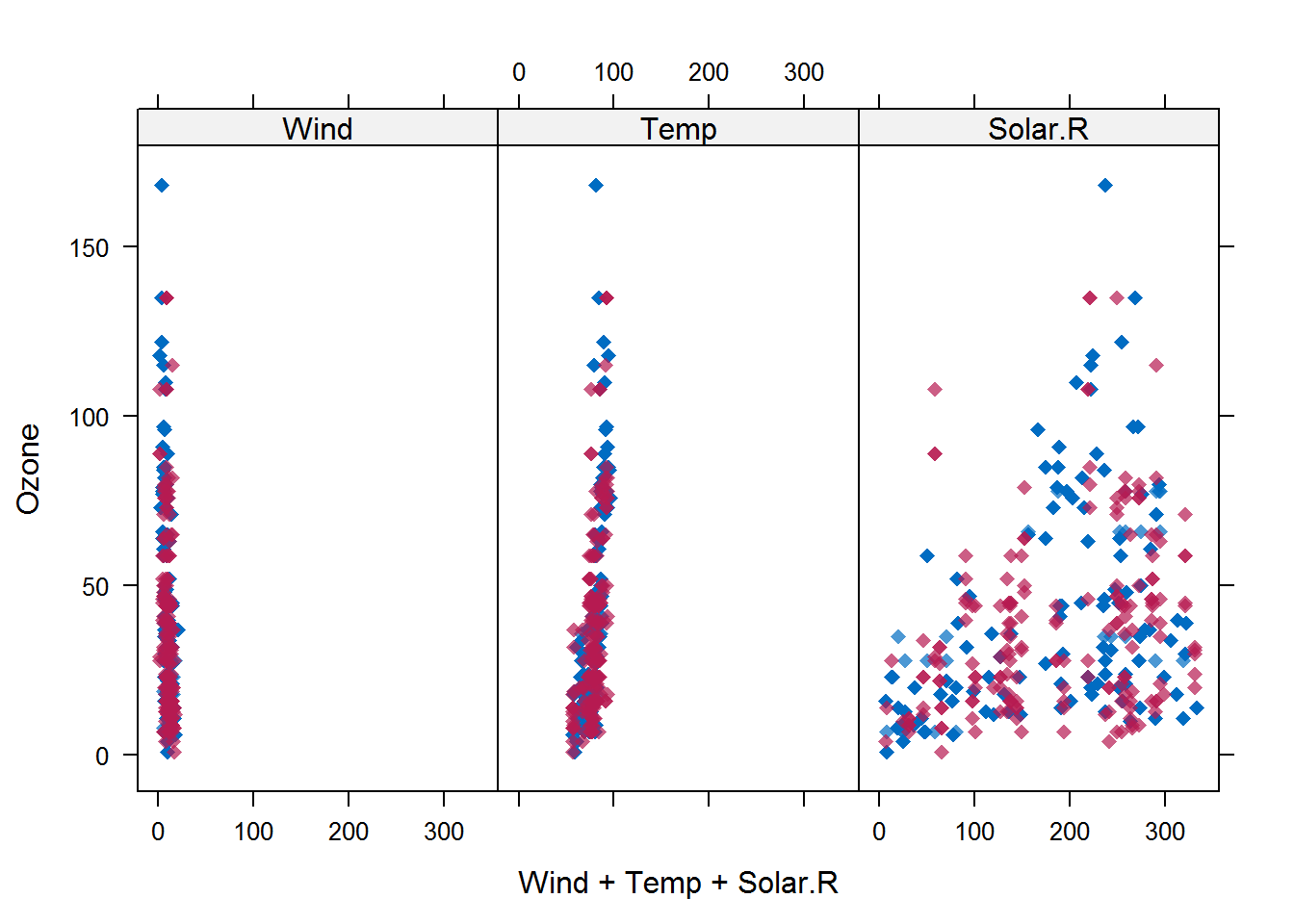We expect to see is that the shape of the magenta points (imputed) matches the shape of the blue ones (observed). The matching shape tells us that the imputed values are indeed “plausible values”.

Another helpful plot is the density plot:

densityplot(tempData)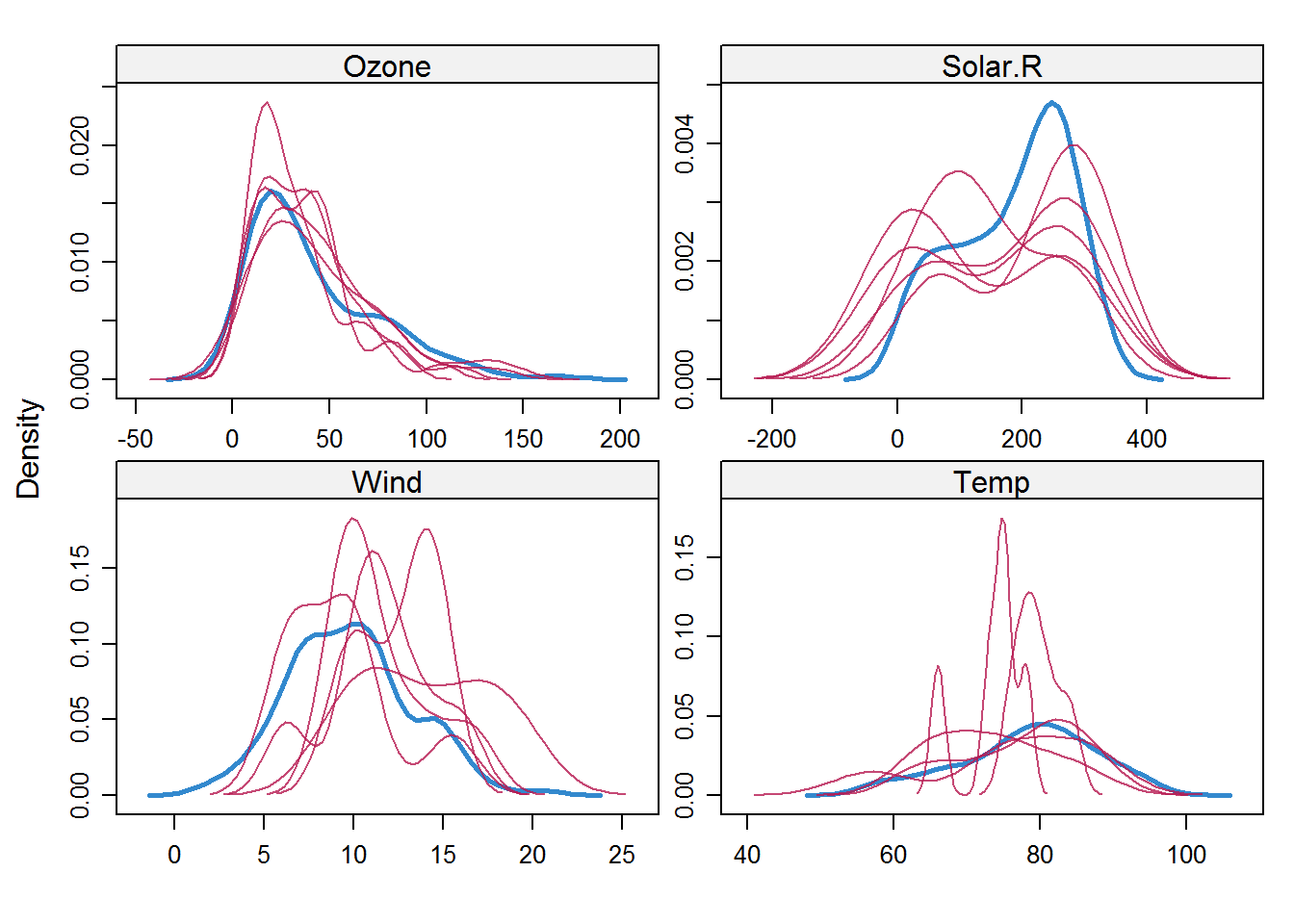The density of the imputed data for each imputed dataset is showed in magenta while the density of the observed data is showed in blue. Again, under our previous assumptions we expect the distributions to be similar.

Another useful visual take on the distributions can be obtained using the stripplot() function that shows the distributions of the variables as individual points:

stripplot(tempData, pch = 20, cex = 1.2)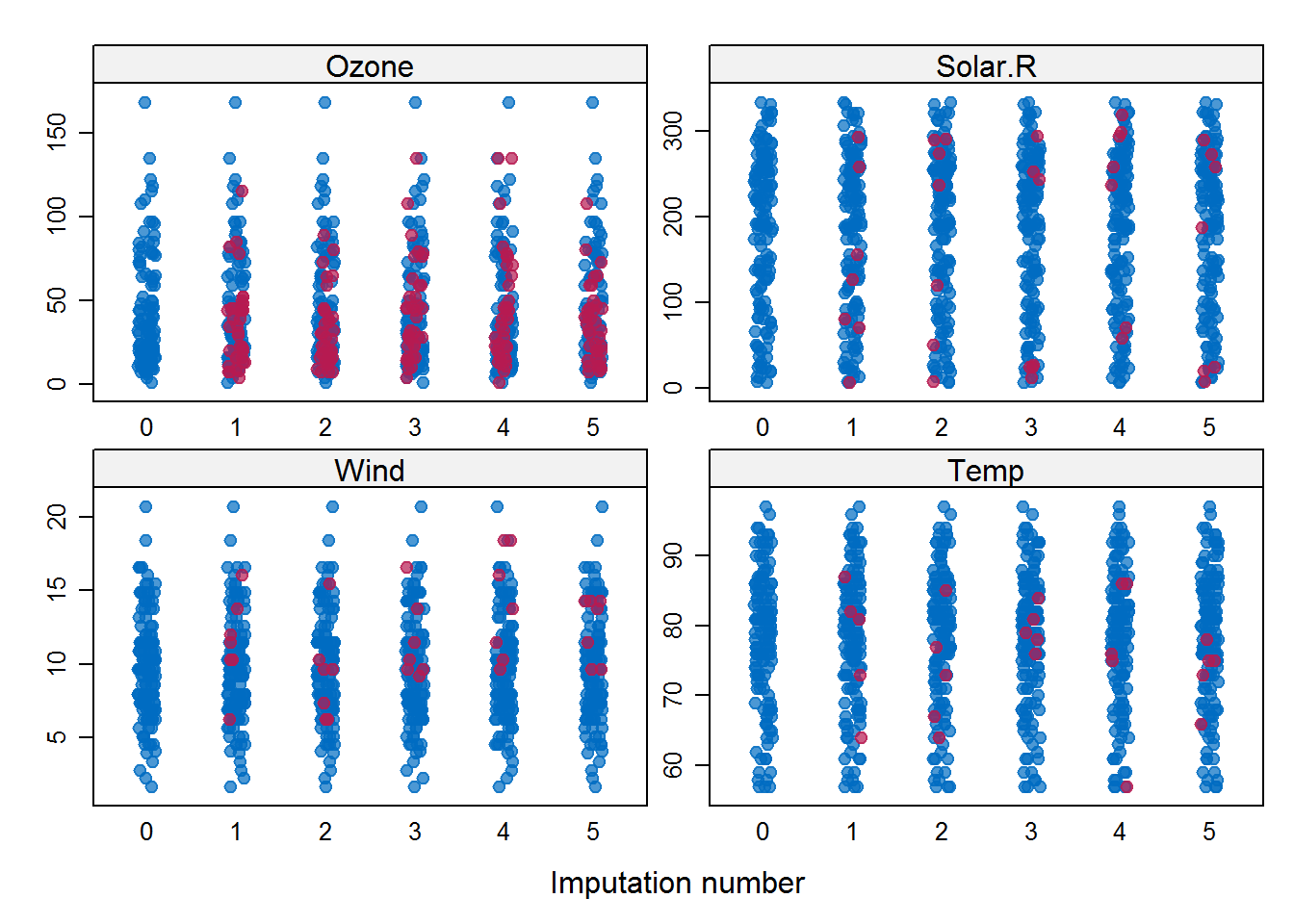## Pooling

Fit a linear model to the data. The MICE package makes it easy to fit a a model to each of the imputed dataset and then pool the results together:

modelFit1 <- with(tempData, lm(Temp ~ Ozone + Solar.R +Wind))
summary(pool(modelFit1))
##                     est          se         t       df     Pr(>|t|)
## (Intercept) 72.83196838 2.984476953 24.403596 43.53891 0.000000e+00
## Ozone        0.16342203 0.024820514  6.584152 97.96154 2.282917e-09
## Solar.R      0.01040475 0.008775564  1.185651 18.75859 2.505626e-01
## Wind        -0.33039675 0.218663873 -1.510980 75.90126 1.349458e-01
##                    lo 95       hi 95 nmis       fmi    lambda
## (Intercept) 66.815350668 78.84858610   NA 0.2698805 0.2370948
## Ozone        0.114166289  0.21267778   37 0.1203435 0.1025658
## Solar.R     -0.007978725  0.02878823    7 0.4632472 0.4089161
## Wind        -0.765912758  0.10511926    7 0.1662491 0.1445654

The variable modelFit1 containts the results of the fitting performed over the imputed datasets. The pool() function pools them all together. The Ozone variable is statistically significant. Note that there are other columns aside from those typical of the lm() model: fmi contains the fraction of missing information while lambda is the proportion of total variance that is attributable to the missing data.

We initialized the mice function with a specific seed, therefore the results are somewhat dependent on our initial choice. To reduce this effect, we can impute a higher number of dataset, by changing the default m = 5 parameter in the MICE() function.

tempData2 <- mice(data, m = 50)
modelFit2 <- with(tempData2, lm(Temp ~ Ozone + Solar.R + Wind))
summary(pool(modelFit2))
##                     est          se         t       df     Pr(>|t|)
## (Intercept) 72.77049386 2.845156817 25.576971 115.5163 0.000000e+00
## Ozone        0.16142418 0.026183862  6.165025 101.3380 1.448599e-08
## Solar.R      0.01226693 0.007185263  1.707235 120.1669 9.036100e-02
## Wind        -0.35035345 0.214336833 -1.634593 123.3348 1.046843e-01
##                    lo 95       hi 95 nmis       fmi    lambda
## (Intercept) 67.135053603 78.40593412   NA 0.1764771 0.1623413
## Ozone        0.109484536  0.21336382   37 0.2426855 0.2278853
## Solar.R     -0.001959189  0.02649305    7 0.1548696 0.1409198
## Wind        -0.774608631  0.07390173    7 0.1400233 0.1261900

We about the same results as before with only Ozone showing statistical significance.